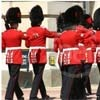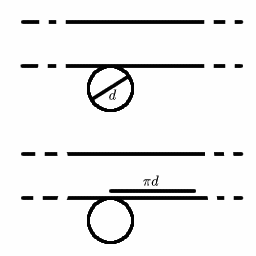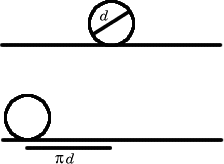#### You may also like### Which Twin Is Older?

A simplified account of special relativity and the twins paradox.### Take a Message Soldier

A messenger runs from the rear to the head of a marching column and back. When he gets back, the rear is where the head was when he set off. What is the ratio of his speed to that of the column?

# Stonehenge

##### Age 16 to 18Challenge Level

Here's a good clear explanation from Jack of Madras College.

Consider the movement of the block relative to the logs:

When the log makes one revolution it travels ${\pi}d$ metres. As the block is in contact with the logs, it moves ${\pi}d$ metres along the horizontal plane.Therefore, the block moves ${\pi}d$ metres relative to the logs.

Now consider the movement of the logs relative to the ground:

When the log makes one revolution it rotates ${\pi}d$ metres. As it is in contact with the ground it moves ${\pi}d$ metres along the horizontal plane.Therefore, the log moves ${\pi}d$ metres relative to the ground.

This means the log moves ${\pi}d$ metres relative to the ground but the block moves ${\pi}d$ metres relative to the logs.

Therefore, the block moves $2{\pi}d$ metres relative to the ground, which is twice as much as the logs.

Thus:- the block moves twice as fast as the logs .